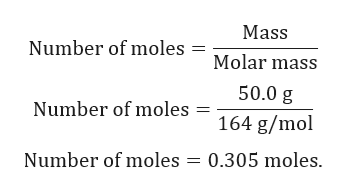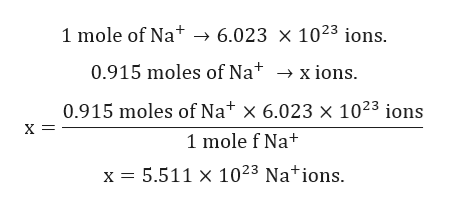# How many cations are there in 50.0 g of sodium phosphate?

Question
340 views

How many cations are there in 50.0 g of sodium phosphate?

check_circle

Step 1

Given:

Mass of compound = 50.0 g.

Formula of sodium phosphate is Na3PO4.

Molar mass of Na3PO4 = 164 g/mol.

Step 2

Calculation for number moles of Na3PO4:help_outlineImage TranscriptioncloseMass Number of moles = Molar mass 50.0 g Number of moles 164 g/mol Number of moles 0.305 moles. fullscreen
Step 3

1 mole of Na3PO4 contains 3 moles of Na+.

Number of moles of Na+ = 3 x 0.305 moles = 0.915 moles...help_outlineImage Transcriptionclose6.023 1023 ions. 1 mole of Na 0.915 moles of Na x ions 0.915 moles of Nat x 6.023 x 1023 ions х- 1 mole f Nat x 5.511 x 1023 Nations fullscreen

### Want to see the full answer?

See Solution

#### Want to see this answer and more?

Solutions are written by subject experts who are available 24/7. Questions are typically answered within 1 hour.*

See Solution
*Response times may vary by subject and question.
Tagged in

### General Chemistry